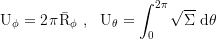## Kerr Metric

English Version
Yukterez
Beiträge: 254
Registriert: Mi 21. Okt 2015, 02:16

### Kerr MetricThis is the english version.Deutschsprachige Version auf kerr.yukterez.net und Yukipedia.Shadow and surfaces of a spinning black hole (a=1), click to enlarge (png). Zoom out: [-], Contours: ƒ, Raytracing Code:Shadow and surfaces of a spinning black hole (a=0.99), Animation parameter: polar angle (θ=1°..90°). Slower:Accretion disk with inner radius ri=isco and outer radius ra=7 around a BH with a=0.95, observer at r=100, θ=70°Retrograde orbit of a particle around a spinning black hole (a=0.95), coordinates: cartesianHere we use natural units of G=M=c=1, so lengths are in GM/c² and times in GM/c³. The metric signature is time-positive (+,-,-,-). a is the spin parameter (for black holes 0≤a≤M), M the mass equivalent of the total energy of the black hole, and Mirr its irreducible mass:Shorthand terms:Covariant metric coeffizients:Contravariant components (superscripted letters are not powers, but indices):The dimensionless spin parameter is a=Jc/G/M². Transformation into cartesian coordinates:Line element in Boyer Lindquist coordinates:Metric tensor (t,r,θ,Ф):With a=0 Boyer Lindquist coordinates reduce to classical Schwarzschild coordinates.With the transformation:where T is a finkelsteinlike time coordinate (radially infalling photons move with dr/dt=1) and ψ the flattened azimuthal angle:the metric in Kerr Schild coordinates (T,r,θ,ψ) is:With a=0 Kerr Schild coordinates reduce to Eddington Finkelstein coordinates.Equations of motion in Boyer Lindquist coordinatesCanonical four-momentum components:Coordinate time by proper time (dt/dτ):First proper time derivative of the radial coordinate (dr/dτ):Derivative of the poloidial (longitudinal) component of motion (dθ/dτ):Derivative of the poloidial angular momentum (dpθ/dτ):Axial (latitudinal) angular momentum:Derivative of the axial component of motion (dФ/dτ):Axial angular momentum derivative (pФ/dτ):Axial component of the angular momentum:Constant of motion, Carter's constant:Constant of motion, Carter k:Constant of motion, total energy:Constant of motion, axial angular momentum:Local 3-velocity component along the r-axis:Local 3-velocity component along the θ-axis:Local 3-velocity component along the Ф-axis:Local 3-velocity, total:For massive testparticles μ=-1 and for photons μ=-0. δ is the inclination angle. With α as the vertical launch anglel the components of the local velocity (relative to a ZAMO) areShapirodelayed and frame dragged velocity as observed at infinity:The radial effective potential which defines the turning points is:Frame-Dragging angular velocity oberserved at infinity (dФ/dt):Delayed Frame-Dragging transverse velocity at the equator of the outer horizon:with the horizons and ergospheres (solution for r at Δ=0 and gtt=0):r and θ dependend delayed Frame-Dragging transverse velocities:at the equatorialen plane at θ=π/2:r und θ dependend local Frame-Dragging transverse velocities (greater than c inside of the ergosphere):at the equatorialen plane at θ=π/2:Cartesian projection of the Frame-Dragging transverse velocity:at the equatorialen plane at θ=π/2:Gravitational time dilation component relative to a ZAMO (dt/dτ):Axial and coaxial radius of gyration:Axial and coaxial circumference:The innermost stable orbit (ISCO) is atwith the shorthand termsFor images and animations see the german version of this site.Simon Tyran aka Симон Тыран @ vk || minds || parler || gab || wikipedia || stackexchange || wolfram